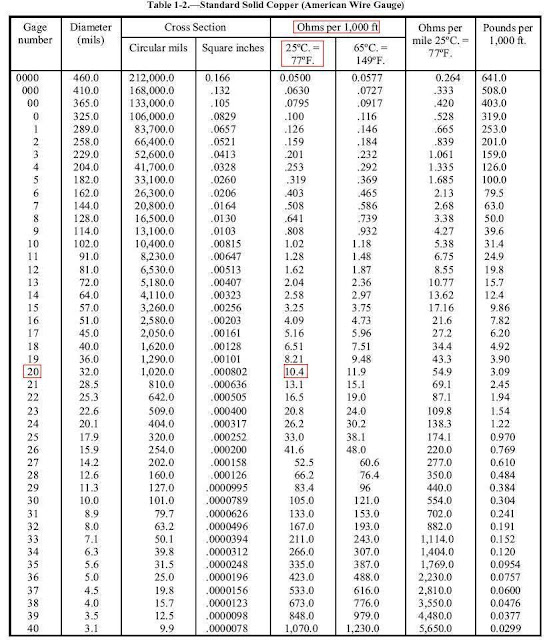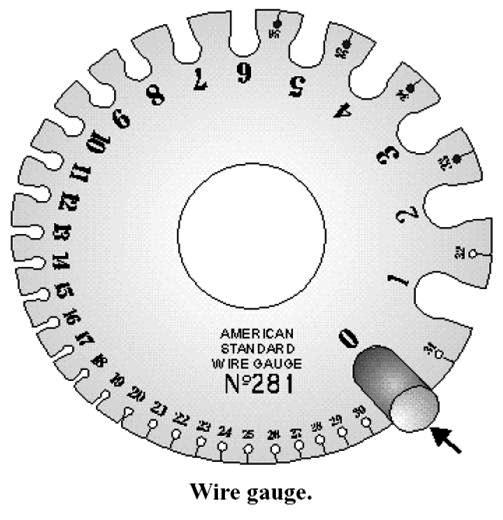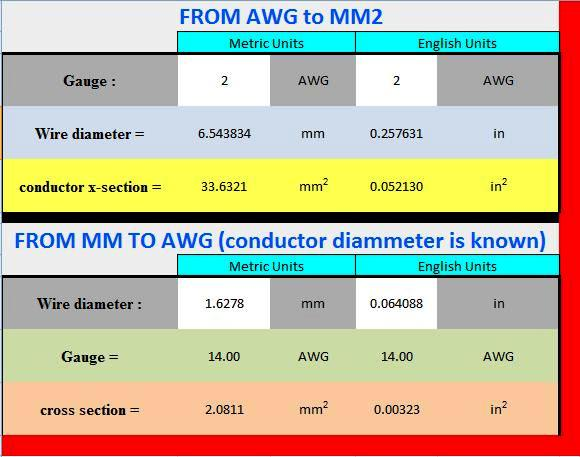### Conductor Ampacity Calculation – Part Two

In Article Conductor Ampacity Calculation – Part One ", I listed the definitions for related terms which were included under the following sections:

1. Conductor/Cable Terms,
2. Conductor/Cable Insulation Materials Terms,
3. Conductor/Cable Tests Terms,
4. Conductor/Cable Manufacturing Process Terms.

Today, I will explain the following points:

1. Measurement Units of Conductors Cross Section Area,
2. Conversions between different Measurement Units of Conductors Cross Section Area,
3. Standard Sizes of Conductors,

1- Measurement Units of Conductors Cross Section Area

1.1 Traditional Measurement Units of Conductors Cross Section Area

As we know that the traditional Measurement Units of Conductors Cross Section Area are:

• Square millimeters (mm2),
• Square inches.

1.2 The measurement unit “SQUARE MIL”:

 Definition: The Mil: is a convenient unit of measurement of the diameter of a conductor Noting that Mil = 0.001 inch = 0.0254 mm Or, Inch = 1000 mils

 Definition: The square Mil: is a unit of measurement used to determine the cross-sectional area of a square or rectangular conductor (views A and B of Fig.1). Also, A square mil : is defined as the area of a square, the sides of which are each 1 mil. , as shown in view A of Fig.2.

 Rule#1: Cross-Sectional Area Of A Square Conductor For Square Conductors, as in View A in fig.1, to obtain the cross-sectional area in circular mils of a square conductor, multiply the dimension of any side of the square by itself. So, Cross-sectional area (A ) in mils = square of Side Length Noting that Side Length must be in mils.Fig (1)

Assume that you have a square conductor with a side dimension of 3 mils. What its cross-sectional area in circular mils?

Solution:

Cross-sectional area (A ) in mils = square of Side Length = 3 x 3 = 9 square mils.

 Rule#2: Cross-Sectional Area Of A Rectangular Conductor For Square Conductors, as in View B in fig.1, to obtain the cross-sectional area in circular mils of a rectangular conductor, multiply the length times the width of the end face of the conductor (side is expressed in mils). So, Cross-sectional area (A ) in mils = Length x Width Noting that Side Length and Width must be in mils.

Example#2:

Assume that one side of the rectangular cross-sectional area is 6 mils and the other side is 3 mils. What its cross-sectional area in circular mils?

Solution:

Cross-sectional area (A) = Length x Width = 6 mils × 3 mils= 18 square mils.

Example#3:

Assume that a conductor is 3/8 inch thick and 4 inches wide. What its cross-sectional area in circular mils?

Solution:

The 3/8 inch can be expressed in decimal form as 0.375 inch. Since 1 mil equals 0.001 inch, the thickness of the conductor will be 0.001 × 0.375, or 375 mils.

Since the width is 4 inches and there are 1,000 mils per inch, the width will be 4 × 1,000, or 4,000 mils.
Cross-sectional area (A) = Length x Width = 375 mils × 4,000 mils= 1,500,000 square mils.

1.3 The measurement unit “CIRCULAR MIL”

 Definition: The circular mil: is the standard unit of measurement of a round wire cross-sectional area (view C of Fig.1). This unit of measurement is found in American and English wire tables. Also, A circular mil: is the area of a circle having a diameter of 1 mil, as shown in view B of Fig.2.

 Important!!! The diameter of a round conductor (wire) used to conduct electricity may be only a fraction of an inch. Therefore, it is convenient to express this diameter in mils to avoid using decimals. For example, the diameter of a wire is expressed as 25 mils instead of 0.025 inch.

 Rule#3: Cross-Sectional Area Of A Round Conductor For Round Conductors, as in View C in fig.1, to obtain the cross-sectional area in circular mils of a round conductor is obtained by squaring the diameter, measured in mils.  So, Cross-sectional area (A) in mils = D2 , D in mils Noting that Diameter D must be in mils.

Example#4:

Assume that a wire having a diameter of 25 mils. What its cross-sectional area in circular mils?

Solution:

Cross-sectional area (A) = D2 = 25 x 25 = 625 circular mils

Comparing Square Mils with Circular Mils for the same Conductor:

Example#5:

A wire having a diameter of 25 mils, compare between its area calculated in Square Mils and in Circular Mils?

Solution:

a- Calculation of conductor area in square mils:

To determine the number of square mils in the same conductor, apply the conventional formula for determining the area of a circle (A = 3.14r2). In this formula, A (area) is the unknown and is equal to the cross-sectional area in square mils, and r is the radius of the circle, or half the diameter (D).

Through substitution, A = 3.14 x (12.5) 2 = 490.625 square mils.

b- Calculation of conductor area in circular mils:

A wire having a diameter of 25 mils has an area of 252, or 625 circular mils.

The cross-sectional area of the wire has 625 circular mils but only 490.625 square mils.

 Important!!! For one conductor (wire or cable), a circular mil represents a smaller unit of area than the square mil.

 Definition: A wire in its usual form is a single slender rod or filament of drawn metal. In large sizes, wire becomes difficult to handle. To increase its flexibility, it is stranded. Strands are usually single wires twisted together in sufficient numbers to make up the necessary cross-sectional area of the cable. So, Stranded Conductor: A conductor composed of individual groups of wires twisted together to form an entire unit.

 Rule#5: Cross-Sectional Area Of A Stranded wire/cable The total area of stranded wire in circular mils is determined by multiplying the area in circular mils of one strand by the number of strands in the cable.  So, Cross-sectional area (A) in mils = N x Cross-sectional area (A) in mils for one stand Where N = number of strands in the cable

1.4 The measurement unit “American Wire Gauge (AWG)”

 Definition: American Wire Gauge (AWG): A standard system used in the United States for expressing wire diameter and designing the size of an electrical conductor based on geometric progression between two conductor sizes, Based on a circular mil system. The American Wire Gage (AWG) is the same as the Brown and Sharpe (BS) Gage.

 Important!!! As the AWG number gets smaller, the wire diameter (gauge) gets larger.

 Rule#6: Expressing wire/cable Size in AWG 1- Wire sizes up to size 4/0 AWG: They are expressed as XX AWG, with XX being the size wire. For example, a wire size expressed as 12 AWG. 2- Conductors larger than 4/0 AWG: They are sized in circular mils, beginning with 250,000 circular mils. For example, a wire size expressed as 250,000-circular-mils. Noting that: 1000 circular-mils = MCM = Kcmil  For example, 250,000-circular-mils conductor was labeled 250 MCM or 250 Kcmil

Example#6:

What is the circular mil area of an 8 AWG solid conductor that has a 0.1285-in. diameter?

Solution:

Diameter (D) in mils = 0.1285 in. x 1000 = 128.5 mils

Cross-sectional area (A) of Round conductor in mils = D2 = 128.5 x 128.5 = 16,512.25 circular mils

Or 16,510 circular mils (rounded off).

2- Conversions between different Measurement Units of Conductors Cross Section Area

 Rule#4: Unit  Conversions Mil = 0.001 inch = 0.0254 mm Inch = 1000 mil = 25.4 mm Square inch = 645.16 mm2 = 1,000,000 square mils Circular mil = 0. 7854 square mil 1000 circular-mils = MCM = Kcmil

Example#7:

A 12-gauge wire has a diameter of 80.81 mils. What is
(1) Its area in circular mils and
(2) Its area in square mils?

Solution:

(1) A = D2 = 80.81 X 80.81 = 6,530 circular mils

(2) A = 0.7854 x 6,530 = 5,128.7 square mils

Example#8:

A rectangular conductor is 1.5 inches wide and 0.25 inch thick. What is
(1) Its area in square mils and
(2) In circular mils? What size of round conductor is necessary to carry the same current as the rectangular bar?

Solution:

(1) 1.5 inches = 1.4 x 1000 = 1,500 mils

0.25 inch = 0.25 x 1000 = 250 mils
A = 1,500 x 250 = 375,000 square mils

(2) To carry the same current, the cross-sectional area of the round conductor must be equal. There are more circular mils than square mils in this area. Therefore:

A = 375,000 / 0.7854 = 477,000 circular mils.

3- Standard Sizes of Conductors

An AWG table for copper wire (solid only) is shown at table #1.Table#1

Notes to table#1:

• The largest wire size shown in the table is 0000 (read "4 naught"), and the smallest is number 40.
• The following sizes can be expressed in other way as follows: 0000 =4/0, 000 = 3/0, 00 = 2/0, 0 = 1/0.
• It shows the diameter in mils, circular mil area, and area in square inches of AWG wire sizes.
• It shows the resistance (ohms) per thousand feet and per mile of wire sizes at specific temperatures.
• It shows the weight of the wire per thousand feet in the last column.

Example#9: (Using table #1)

You are required to run 2,000 feet of AWG 20 solid copper wire for a new piece of equipment. The temperature where the wire is to be run is 25º C (77º F). How much resistance will the wire offer to current flow?

Solution:

In table#1, under the gauge number column, find size AWG 20. Now read across the columns until you reach the "ohms per 1,000 feet for 25º C (77º F)" column.

You will find that the wire will offer 10.4 ohms of resistance to current flow. Since we are using 2,000 feet of wire, multiply by 2.
Resistance of 1,000 feet for 25º C (77º F) = 10.4 ohms

So, Resistance of 2,000 feet = 10.4 ohms x 2 = 20.8 ohms

 Important!!! An American Standard Wire Gauge (fig.3) is used to measure wires ranging in size from number 0 to number 36. To use this gauge, insert the wire to be measured into the smallest slot that will just accommodate the bare wire. The gauge number on that slot indicates the wire size. The front part of the slot has parallel sides, and this is where the wire measurement is taken. It should not be confused with the larger semicircular opening at the rear of the slot. The rear opening simply permits the free movement of the wire all the way through the slot.Fig (3)

Table#2 introduces copper wire AWG sizes (solid and stranded) as follows:

 AWG MM2 AWG MM2 30 0.05 6 16 28 0.08 4 25 26 0.14 2 35 24 0.25 1 50 22 0.34 1/0 55 21 0.38 2/0 70 20 0.50 3/0 95 18 0.75 4/0 120 17 1.0 300MCM 150 16 1.5 350MCM 185 14 2.5 500MCM 240 12 4.0 600MCM 300 10 6.0 750MCM 400 8 10.0 1000MCM 500

Table#2

Or you can use the following excel sheet converter from AWG to MM2 or vice versa.In the next Article, I will continue explaining the Conductor Ampacity calculations. Please, keep following.

#### 1 comment:

1.Hi, I would like to know if is possible to measure the cross-section of long cylindrical products made of conductive material, that means, I am building a cable and I would measure the diameter of this at same time I am building, Is any device developed for measuring it?

I was reading a lot about it in the net but I didn't find specific information.

Thanks